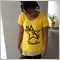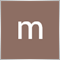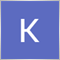# Coding help - page 78519

Hello people.  May I know what I need to make the code draws box and print text only ONCE after the candle pattern detected closed?

```//+------------------------------------------------------------------+
//| Custom indicator iteration function                              |
//+------------------------------------------------------------------+
int OnCalculate(const int rates_total,
const int prev_calculated,
const datetime &time[],
const double &open[],
const double &high[],
const double &low[],
const double &close[],
const long &tick_volume[],
const long &volume[],
{
//---code start
int counted_bars=IndicatorCounted();
//---check for possible errors
if (counted_bars<0) return(-1);
//---last counted bar will be recounted
if (counted_bars>0) counted_bars--;
int limit=Bars-counted_bars;
for(int i = limit-1; i >= 0; i--)
{
if (i >= MathMin(Maxbars-1, rates_total-1-50)) continue;

double high1  = High[i];
double low1   = Low[i];
double open1  = Open[i];
double close1 = Close[i];
double high2  = High[i+1];
double low2   = Low[i+1];

Bar1[i] = EMPTY;
Bar2[i] = EMPTY;
Bar3[i] = EMPTY;
Bar4[i] = EMPTY;
int sigtf=0;
if(_Period==1440 || _Period==43200){sigtf=6;}
else{sigtf=5;}
int sigbox=0;
//double   priceopen = iOpen(NULL,0,1);
//double   priceclose = iClose(NULL,0,1);
if (high1>high2 && low1<low2 && close1>open1)
{
Bar1[i] = High[i];
Bar2[i] = Low[i];
Bar3[i] = Open[i];
Bar4[i] = Close[i];
sigbox = 1;
Print("test 1");
}

if(sigbox==1)
{
sigbox=0;
DrawBox("Area",i,Time[i],Bar1[i],Time[i]+((_Period*sigtf)*60),Bar2[i],clrGainsboro);
}
else
{
sigbox=0;
}
}
ChartRedraw(0);
//---code end
return(rates_total);
}

void DrawBox(string bxname, int i, datetime time1, double price1,  datetime time2, double price2, color bxcolor)
{
string objname = objref+bxname+(string)i;
ObjectDelete(objname);
ObjectCreate(0,objname,OBJ_RECTANGLE,0,time1,price1,time2,price2);
ObjectSet(objname, OBJPROP_COLOR, bxcolor);
ObjectSet(objname, OBJPROP_STYLE, STYLE_SOLID);
ObjectSet(objname, OBJPROP_WIDTH, 0);
ObjectSet(objname, OBJPROP_FILL, true);
ObjectSet(objname, OBJPROP_BACK, false);
ObjectSet(objname, OBJPROP_SELECTABLE, false);
ObjectSet(objname, OBJPROP_HIDDEN, true);
}```

Files:8

how to make a mq4 horizontal line with your own value. pleasee110

```#region Using declarations
using System;
using System.ComponentModel;
using System.Diagnostics;
using System.Drawing;
using System.Drawing.Drawing2D;
using System.Xml.Serialization;
#endregion

// This namespace holds all indicators and is required. Do not change it.
{
/// <summary>
/// Enter the description of your new custom indicator here
/// </summary>
[Description("Enter the description of your new custom indicator here")]
public class NPSqueeze : Indicator
{
#region Variables
// Wizard generated variables
private int length = 20; // Default setting for Length
private double bBDev = 2; // Default setting for BBDev
private double kCDev = 1.5; // Default setting for KCDev
// User defined variables (add any user defined variables below)
#endregion

/// <summary>
/// This method is used to configure the indicator and is called once before any bar data is loaded.
/// </summary>
protected override void Initialize()
{
Overlay                             = false;
}

/// <summary>
/// Called on each bar update event (incoming tick)
/// </summary>
protected override void OnBarUpdate()
{
// Use this method for calculating your indicator values. Assign a value to each
// plot below by replacing 'Close' with your own formula.
double value = Bollinger(bBDev,length).Upper-KeltnerChannel(kCDev,length).Upper ;
Squeeze.Set(value);
}

#region Properties
[Browsable(false)]      // this line prevents the data series from being displayed in the indicator properties dialog, do not remove
[XmlIgnore()]           // this line ensures that the indicator can be saved/recovered as part of a chart template, do not remove
public DataSeries Squeeze
{
get { return Values; }
}

[Description("BB and KC average length")]
[GridCategory("Parameters")]
public int Length
{
get { return length; }
set { length = Math.Max(1, value); }
}

[Description("BB deviations")]
[GridCategory("Parameters")]
public double BBDev
{
get { return bBDev; }
set { bBDev = Math.Max(0, value); }
}

[Description("KC deviation in ATR's")]
[GridCategory("Parameters")]
public double KCDev
{
get { return kCDev; }
set { kCDev = Math.Max(0, value); }
}
#endregion
}
}

#region NinjaScript generated code. Neither change nor remove.
// This namespace holds all indicators and is required. Do not change it.
{
public partial class Indicator : IndicatorBase
{
private NPSqueeze[] cacheNPSqueeze = null;

private static NPSqueeze checkNPSqueeze = new NPSqueeze();

/// <summary>
/// Enter the description of your new custom indicator here
/// </summary>
/// <returns></returns>
public NPSqueeze NPSqueeze(double bBDev, double kCDev, int length)
{
return NPSqueeze(Input, bBDev, kCDev, length);
}

/// <summary>
/// Enter the description of your new custom indicator here
/// </summary>
/// <returns></returns>
public NPSqueeze NPSqueeze(Data.IDataSeries input, double bBDev, double kCDev, int length)
{
if (cacheNPSqueeze != null)
for (int idx = 0; idx < cacheNPSqueeze.Length; idx++)
if (Math.Abs(cacheNPSqueeze[idx].BBDev - bBDev) <= double.Epsilon && Math.Abs(cacheNPSqueeze[idx].KCDev - kCDev) <= double.Epsilon && cacheNPSqueeze[idx].Length == length && cacheNPSqueeze[idx].EqualsInput(input))
return cacheNPSqueeze[idx];

lock (checkNPSqueeze)
{
checkNPSqueeze.BBDev = bBDev;
bBDev = checkNPSqueeze.BBDev;
checkNPSqueeze.KCDev = kCDev;
kCDev = checkNPSqueeze.KCDev;
checkNPSqueeze.Length = length;
length = checkNPSqueeze.Length;

if (cacheNPSqueeze != null)
for (int idx = 0; idx < cacheNPSqueeze.Length; idx++)
if (Math.Abs(cacheNPSqueeze[idx].BBDev - bBDev) <= double.Epsilon && Math.Abs(cacheNPSqueeze[idx].KCDev - kCDev) <= double.Epsilon && cacheNPSqueeze[idx].Length == length && cacheNPSqueeze[idx].EqualsInput(input))
return cacheNPSqueeze[idx];

NPSqueeze indicator = new NPSqueeze();
indicator.BarsRequired = BarsRequired;
indicator.CalculateOnBarClose = CalculateOnBarClose;
#if NT7
indicator.ForceMaximumBarsLookBack256 = ForceMaximumBarsLookBack256;
indicator.MaximumBarsLookBack = MaximumBarsLookBack;
#endif
indicator.Input = input;
indicator.BBDev = bBDev;
indicator.KCDev = kCDev;
indicator.Length = length;
indicator.SetUp();

NPSqueeze[] tmp = new NPSqueeze[cacheNPSqueeze == null ? 1 : cacheNPSqueeze.Length + 1];
if (cacheNPSqueeze != null)
cacheNPSqueeze.CopyTo(tmp, 0);
tmp[tmp.Length - 1] = indicator;
cacheNPSqueeze = tmp;
return indicator;
}
}
}
}

// This namespace holds all market analyzer column definitions and is required. Do not change it.
{
public partial class Column : ColumnBase
{
/// <summary>
/// Enter the description of your new custom indicator here
/// </summary>
/// <returns></returns>
[Gui.Design.WizardCondition("Indicator")]
public Indicator.NPSqueeze NPSqueeze(double bBDev, double kCDev, int length)
{
return _indicator.NPSqueeze(Input, bBDev, kCDev, length);
}

/// <summary>
/// Enter the description of your new custom indicator here
/// </summary>
/// <returns></returns>
public Indicator.NPSqueeze NPSqueeze(Data.IDataSeries input, double bBDev, double kCDev, int length)
{
return _indicator.NPSqueeze(input, bBDev, kCDev, length);
}
}
}

// This namespace holds all strategies and is required. Do not change it.
{
public partial class Strategy : StrategyBase
{
/// <summary>
/// Enter the description of your new custom indicator here
/// </summary>
/// <returns></returns>
[Gui.Design.WizardCondition("Indicator")]
public Indicator.NPSqueeze NPSqueeze(double bBDev, double kCDev, int length)
{
return _indicator.NPSqueeze(Input, bBDev, kCDev, length);
}

/// <summary>
/// Enter the description of your new custom indicator here
/// </summary>
/// <returns></returns>
public Indicator.NPSqueeze NPSqueeze(Data.IDataSeries input, double bBDev, double kCDev, int length)
{
if (InInitialize && input == null)
throw new ArgumentException("You only can access an indicator with the default input/bar series from within the 'Initialize()' method");

return _indicator.NPSqueeze(input, bBDev, kCDev, length);
}
}
}```

Please i need help with converting a Ninja indicator into MQL4, MT4.

Rgds.11

Afghan123 #:
ok
Requisition for a code11

Thanks for the update11

Pls how can I get my indicator 411

Good day coders,

I have developed a MATLAB code for prediction of forex pairs price for next day. Now, I need an expert in mql4 which can read a .csv file: contains date, time, symbol and signal(buy=1, sell=-1 and 0=nothing) with constant SL,TP and LOTS.

I will be appreciated if you provide it for me.

Thanks Maryam.347